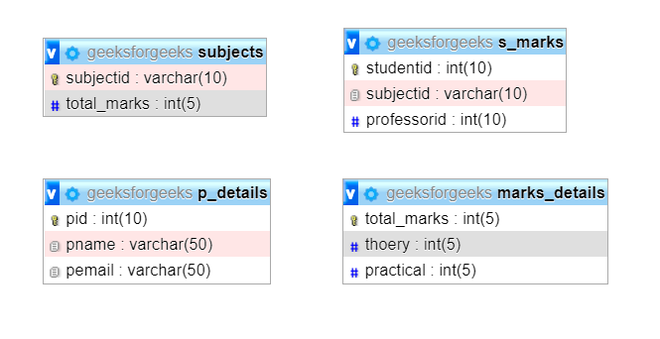Related Articles

# Joining 4 Tables in SQL

• Difficulty Level : Expert
• Last Updated : 01 Sep, 2021

The purpose of this article is to make a simple program to Join two tables using Join and Where clause in SQL. Below is the implementation for the same using MySQL. The prerequisites of this topic are MySQL and the installment of Apache Server on your computer.

Introduction :
In SQL, a query is a request with some instruction such that inserting, reading, deleting, and updating, etc. record from the database. This data can be used for various purposes like Training a model, finding the patterns in the data, etc. Here, we will discuss the approach for Joining 4 Tables in SQL and will implement using SQL query for each table for better understanding.

Approach :
Here, we will discuss the approach and steps to implement Joining 4 Tables in SQL. So, let’s start by creating a Database.

Step-1: Create a database –
Here first, we will create the database using SQL query as follows.

`CREATE DATABASE geeksforgeeks;`

Step-2: Use the database –
Now, we will use the database using SQL query as follows.

`USE geeksforgeeks;`

Step-3: Creating table1 –
Create a table 1, name as s_marks using SQL query as follows.

```CREATE TABLE s_marks
(
studentid int(10) PRIMARY KEY,
subjectid VARCHAR(10),
professorid int(10)
);```

Step-4: Creating table2 –
Create a table2 for the professor details as p_details using SQL query as follows.

```CREATE TABLE p_details
(
pid int(10) PRIMARY KEY,
pname VARCHAR(50),
pemail VARCHAR(50)
);```

Step-5: Creating table3 –
Create a table for subjects as subjects using SQL query as follows.

```CREATE TABLE subjects
(
subjectid VARCHAR(10) PRIMARY KEY,
total_marks INT(5)
);```

Step-6: Creating table4 –
Create a table for the subject marks details using SQL query as follows.

```CREATE TABLE marks_details
(
total_marks INT(5) PRIMARY KEY,
theory INT(5),
practical INT(5)
);```

Output :
The output of tables as follows.Step-7: Inserting data :
Insert some data in the above-created tables using SQL query as follows.

Insert into s_marks –

```INSERT INTO `s_marks` (`studentid`, `subjectid`, `professorid`) VALUES ('1', 'KCS101', '1');
INSERT INTO `s_marks` (`studentid`, `subjectid`, `professorid`) VALUES ('2', 'KCS102', '2');```

Insert into p_details –

```INSERT INTO `p_details` (`pid`, `pname`, `pemail`) VALUES ('1', 'Devesh', 'geeks@abc.com');
INSERT INTO `p_details` (`pid`, `pname`, `pemail`) VALUES ('2', 'Aditya', 'for@abc.com');```

Insert into subjects –

```INSERT INTO `subjects` (`subjectid`, `total_marks`) VALUES ('KCS101', '100');
INSERT INTO `subjects` (`subjectid`, `total_marks`) VALUES ('KCS102', '150');```

Insert into marks_details –

```INSERT INTO `marks_details` (`total_marks`, `theory`, `practical`) VALUES ('100', '70', '30');
INSERT INTO `marks_details` (`total_marks`, `theory`, `practical`) VALUES ('150', '100', '50');```

Step-8: Verifying and joining tables –
Run the query to find out the ID, professor name of a student whose subject’s practical marks are 50 as follows.

```SELECT s_marks.studentid, p_details.pname FROM s_marks
JOIN subjects ON s_marks.subjectid = subjects.subjectid
JOIN marks_details ON subjects.total_marks = marks_details.total_marks
JOIN p_details ON p_details.pid = s_marks.professorid
WHERE marks_details.practical = '50';```

Output :

Attention reader! Don’t stop learning now. Learn SQL for interviews using SQL Course  by GeeksforGeeks.

My Personal Notes arrow_drop_up
Recommended Articles
Page :